# Advanced Introduction to C++, Scientific Computing and Machine Learning

Claudius Gros, SS 2023

Institut for Theoretical Physics
Goethe-University Frankfurt a.M.

# variational inference

Graves (2011)
Practical variational inference for neural networks

### conditional probabilities

• $\mathcal{D}\$ : dataset with elements $(\mathbf{x},\mathbf{y})$,
$\mathbf{x}\$ : input
$\mathbf{y}\$ : output
• $\mathbf{w}\$ : network parameters (weights)
• $P(\mathcal{D}|\mathbf{w})\$ : conditional probability for data $\mathcal{D}$, for a given configuration of $\mathbf{w}$
:: both $\mathbf{x}$ and $\mathbf{y}$ are given for supervised learning

### accuracy objective

• $L^N(\mathbf{w},\mathcal{D})\$ : network loss function
$$L^N(\mathbf{w},\mathcal{D}) = -\log\big(P(\mathcal{D}|\mathbf{w})\big) = - \sum_{(\mathbf{x},\mathbf{y})\in\mathcal{D}} \log\big(P(\mathbf{y}|\mathbf{x},\mathbf{w})\big)$$
• is minimal if there is one $\mathbf{y}$ for every $\mathbf{x}$
:: entropy is positive definite, vanishing in the absence of noise

### variational inference

• $P(\mathbf{w}|\alpha)\$ : prior distribution of weights (before training), depending on parameter $\alpha$
• $P(\mathbf{w}|\mathcal{D},\alpha)\$ : posterior weight distribution (given training data $\mathcal{D}$)
:: not tractable
• $P(\mathbf{w}|\mathcal{D},\alpha)$ is approximated by
$Q(\mathbf{w}|\beta)$, determined variationally, viz by minimizing a given objective function
• $\mathcal{F}\$ : variational free energy
$$\mathcal{F} = - \left\langle \log\left[ \frac{P(\mathcal{D}|\mathbf{w})P(\mathbf{w}|\alpha)}{Q(\mathbf{w}|\beta)} \right]\right\rangle_{\mathbf{w}\sim Q(\beta)}$$

### Kullback Leibler divergence

$$\mathcal{F} = \left\langle L^N(\mathbf{w},\mathcal{D}) \right\rangle_{\mathbf{w}\sim Q(\beta)} + D_{\rm KL}\big(Q(\beta)\parallel P(\alpha)\big)$$
• $\langle L^N(\mathbf{w},\mathcal{D}) \rangle_{\mathbf{w}\sim Q(\beta)}\$ : parametrized by $Q(\beta)$, instead of $\mathbf{w}$
• $D_{\rm KL}(Q(\beta)\parallel P(\alpha)) \$ : Kullback-Liebler divergence ('distance')
:: positive definite
:: $D_{\rm KL}\equiv 0\$ if and only if $Q(\beta)$ and $P(\alpha)$ identical
:: converged if posterior $\equiv$ prior

### variational distributions

• minimization procedure possible for a given parametrization of $Q(\mathbf{w},\beta)$
• Gaussian: mean $\mu_i$ and standard deviation $\sigma_i$ for parameter $w_i$
• same parametrization for prior $P(\mathbf{w}|\alpha)$

### inference

• result is a distribution, parametrized by $(\mu_i,\sigma_i)$,
for network parameters: not a single value
• set of $(\mu_i,\sigma_i)$ updated with every new observation,
:: viz with every new data $(\mathbf{x},\mathbf{y})$
:: inference: updating of a distribution with new observations
• next step: start posterior as new prior
:: $(\mu_i,\sigma_i)$ are now the parameters of the prior

# variational autoencoder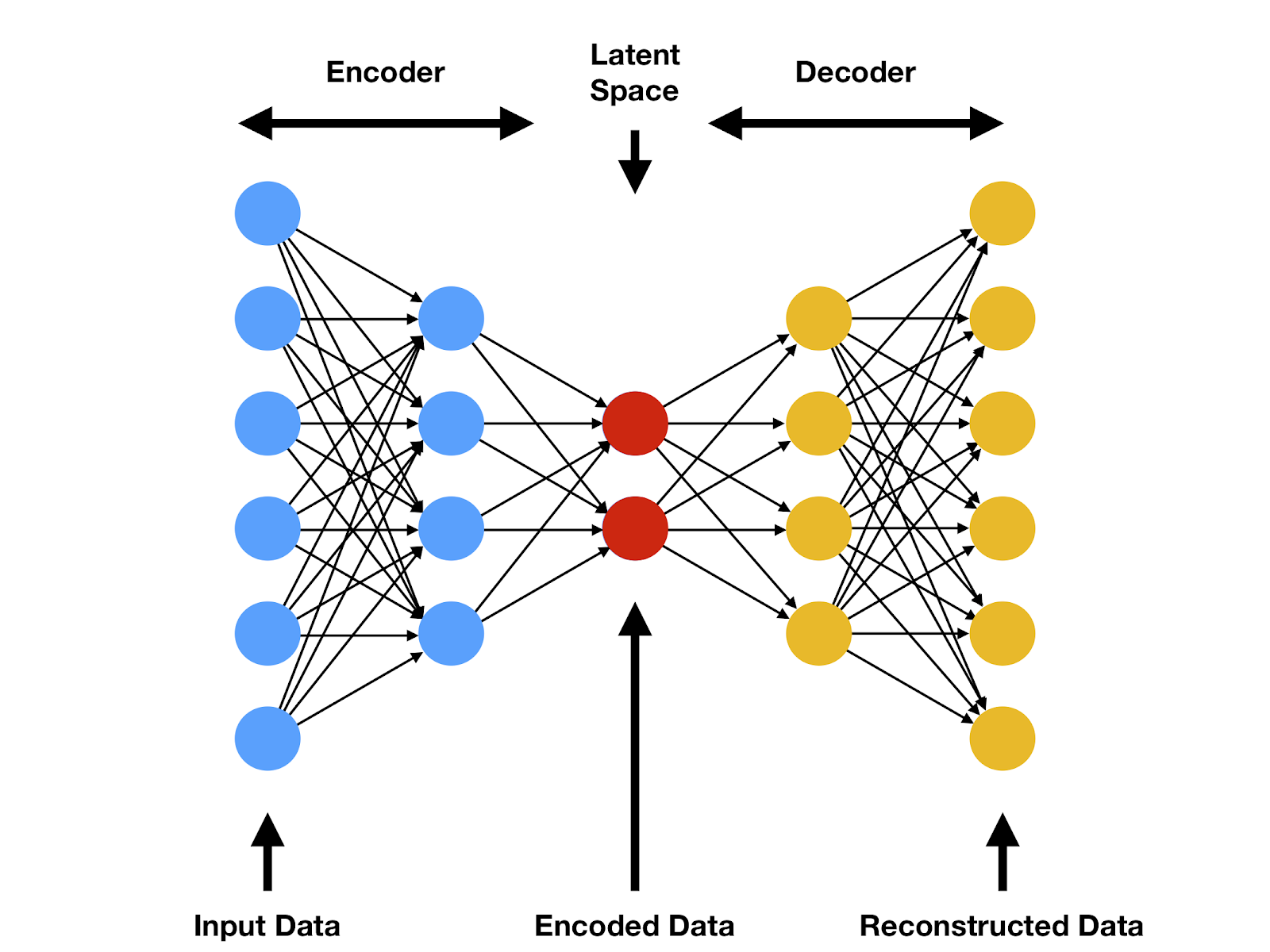• latent space ('encoded space')
• for normal autoencoder
:: not structured, just a bottleneck
• for variational autoencoder
:: maximal information
• maximize information when encoding
• minimized reconstruction error when decoding
• $\mathbf{x}\$ : input
$\mathbf{z}\$ : latent state
$\mathbf{y}\$ : output

### probabilistic network representations

• $Q(\mathbf{w}|\beta)\$ : encoder
$Q^\prime(\mathbf{w}^\prime|\beta^\prime)\$ : decoder
:: probabilities to generated networks parameters $\mathbf{w}\,/\,\mathbf{w}^\prime$
:: encoded by variational parameters $\beta\,/\,\beta^\prime$ (Gaussians)

### accuracy target

• $P(\mathbf{y}|\mathbf{z},\mathbf{w}^\prime)\$ : probability to find $\mathbf{y}$ for given $\mathbf{z}$ and $\mathbf{w}^\prime$
$$\mathcal{F}_{\mathrm{accuracy}} = - \sum_{(\mathbf{x},\mathbf{y})\in\mathcal{D}} \log\big(P(\mathbf{y}|\mathbf{z},\mathbf{w}^\prime)\big)$$
• $\mathbf{y}\hat{=}\mathbf{x}\$ for autoencoder
• minimal if there is an $\mathbf{y}$ for every $\mathbf{z}$

### information maximization

• variational inference between input and latent space
• posterior and prior (both variational)
$$\mathcal{F}_{\mathrm{information}} = D_{\mathrm{KL}} \big(P_{\mathrm{posterior}} \parallel P_{\mathrm{prior}} \big)$$
• with
$$P_{\mathrm{prior/posterior}} = P(\mathbf{z}|\mathbf{x},\mathbf{w})\Big|_{\mathrm{before/after\ updating}}$$

# natural language processing (NLP)[Smerity]

### encoder-decoder architectures for NLP

• encoder
$\mathbf{h}_{t} = \mathbf{f}(\mathbf{h}_{t-1}, \mathbf{x}_t)$
$\mathbf{h}_{t}\$ : hidden layer state
$\mathbf{x}_{t}\$ : input (embedded words)
• decoder
every layer makes a prediction,
receiving previous predictions as input
$\mathbf{s}_{t} = \mathbf{g}(\mathbf{s}_{t-1}, \mathbf{y}_{t-1})$
$\mathbf{s}_{t}\$ : hidden layer state
$\mathbf{y}_{t}\$ : predicted word (linear classifier, softmax)

### word embedding

• preprocessing: word $\to$ vector
• consider inter-word correlations
:: related words are closer in vector space

### variants

• apply to
• variational encoders, decoders, and/or
• recurrent neural networks (RNN)
• normal units / long short-term units
• state-of-the-art until 2014-2017

# semantic correlations

If the universe has an explanation of its existence,
that explanation is God.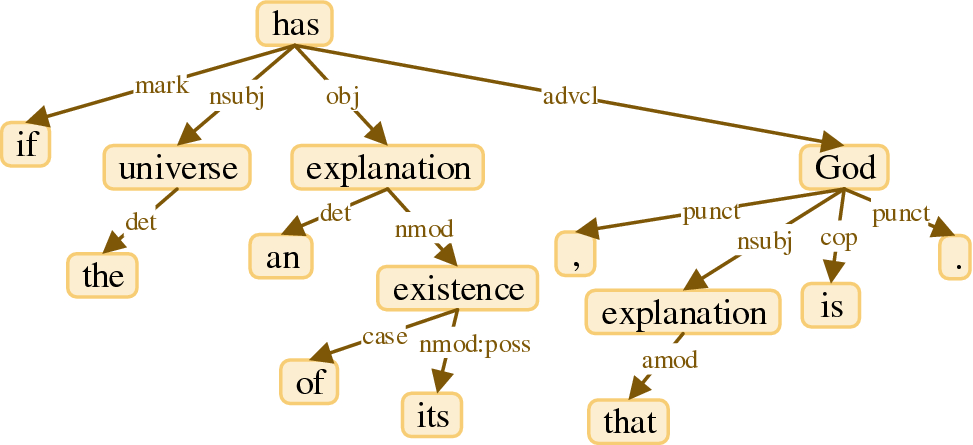• posibly long-distance semantic correlations
• state-of-the-art: attention

# query, key & value

• $\sim0.75$ word -- tokens
$\sim 5\cdot10^4$:   vocabulary size
• activity $\ \mathbf{x}$: current state
• three matrices (entries adapted via backpropagation)
$\hat{Q}\$: query
$\hat{K}\$: key
$\hat{V}\$: value
• $\mathbf{Q}$, $\mathbf{K}$, $\mathbf{V}$ vectors
$$\mathbf{Q} = \hat{Q}\cdot\mathbf{x},\qquad \mathbf{K} = \hat{K}\cdot\mathbf{x},\qquad \mathbf{V} = \hat{V}\cdot\mathbf{x},\qquad$$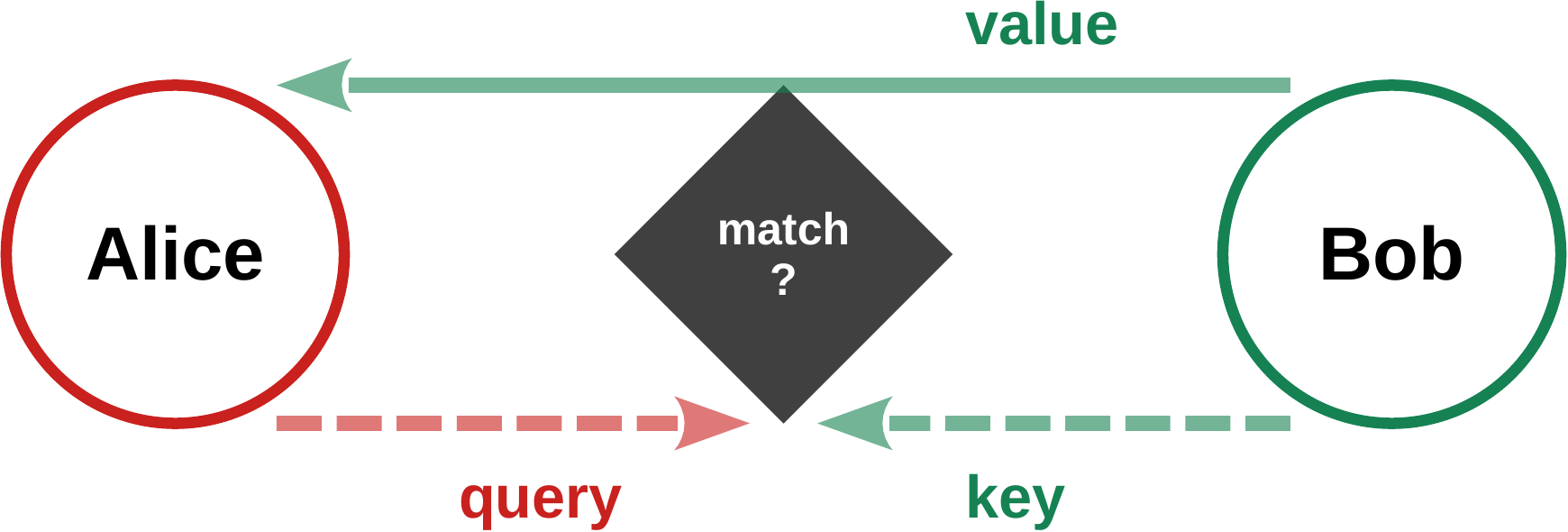### Alice: should I pay attention to Bob?

• databank query formalism
• Alice sends its query $\ \mathbf{Q}_A\$ to Bob
• Bob compares $\ \mathbf{Q}_A\$ with its key $\ \mathbf{K}_B$
• if there is a match, sends back its value $\ \mathbf{V}_B$
• content-based attention via similarity
• self attention: between words of a sentence

# dot-product attention• scanning keys for similarity with query
$\mathbf{K}_j \$ : key of object
$\mathbf{V}_j \$ : value of object
$\mathbf{Q}_{\phantom{j}} \$ : query
$$\mathbf{a} = \sum_j \alpha_j\mathbf{V}_j, \quad\qquad \alpha_{j} = \frac{\mathrm{e}^{e_{j}}}{\sum_i\mathrm{e}^{e_{i}}} \quad\qquad e_{j} = \mathbf{Q}\cdot\mathbf{K}_{j}$$
• $\mathbf{a}\$ : attention vector
:: activity of query token
:: output of attention layer
• softmax (Boltzman distribution) superposition of values
• similarity: scalar product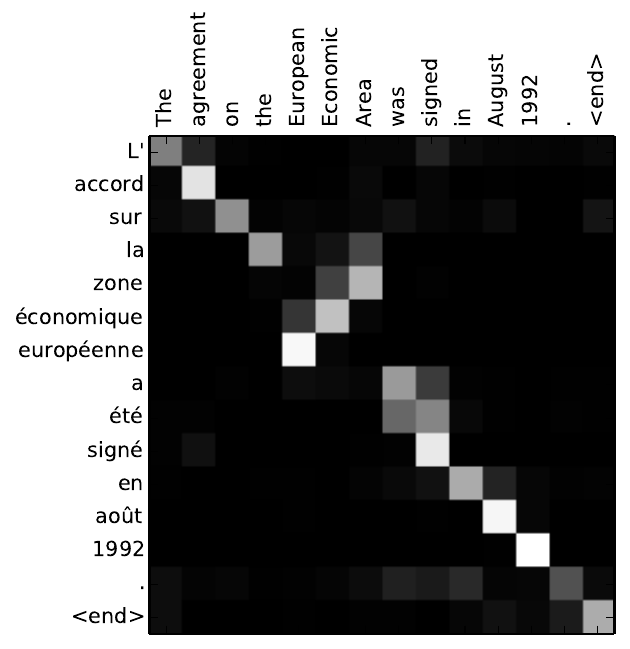# transformer block$$\begin{array}{ccccccc} \fbox{\phantom{\big|}\mathrm{input}\phantom{\big|}} &\to& \fbox{\phantom{\big|}\mathrm{attention}\phantom{\big|}} &\to& \fbox{\phantom{\big|}\mathrm{normalization}\phantom{\big|}} & & \\[0.5ex] &\to& \fbox{\phantom{\big|}\mathrm{feed forward}\phantom{\big|}} &\to& \fbox{\phantom{\big|}\mathrm{normalization}\phantom{\big|}} &\to& \fbox{\phantom{\big|}\mathrm{output}\phantom{\big|}} \end{array}$$
• input sentence
:: embedded sequence of words (tokens)
• residual connections around attention layer are essential
• MLP: linear feedword layer:
• output of block $\ \equiv\$ input of next block

• $h\$ attention blocks in parallel ($h=8$)
:: every token generates $\ h \$ Q/K/V matrices
• allows for context specific attention

# skip connections[Smerity]

### skip/residual connections

• add identity in parallel to hidden layer
$\mathbf{Layer}(\mathbf{x}) \ \to \ \mathbf{x}+\mathbf{Layer}(\mathbf{x})$
$\mathbf{Norm}\Big(\mathbf{x}+\mathbf{Layer}(\mathbf{x})\Big)$

• helps with vanishing gradient problem
when backprogating learning signals
during the training of deep networks
• if layer is superfluous, training can ignore it
• allows to add (self-) attention layers (transformer model)

# positional encoding

• NLP before transformer (2017)
:: encoder / decoder with RNN and LTSM
• NLP with transformer
:: no RNN, no LTSM; good for parallelization, GPUs
:: uses self-attention with positional encoding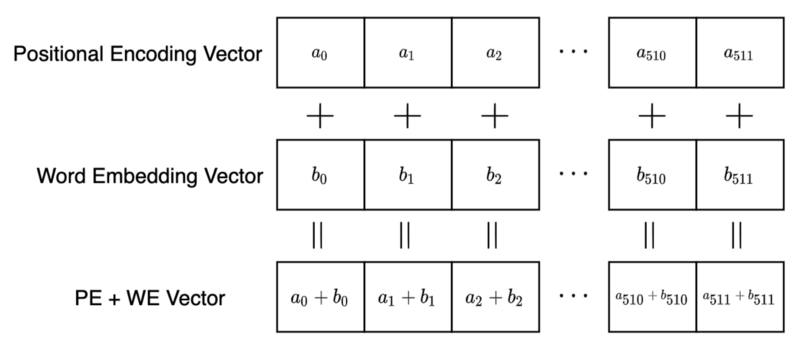### position of words in a sentence

• system needs position information when
• for every word:
$\mathbf{P}_e\$ : positional encoding vector
$\mathbf{W}_e\$ : word embedding vector
• transformer architecture
$\mathbf{P}_e$ and $\mathbf{W}_e$ have identical dimension
$d_{\mathrm{model}}=512 \$ : model dimension
• encoder input:
$\mathbf{P}_e + \mathbf{W}_e\$ : vector sum (times number of words)

### discrete fourier analysis

• many types of positional encodings possible
transfomer uses fourier components
• $L_s\$ : length of input sentence
$k\$ : word position
$d_{\rm model}\$ : embedding dimension
$i\in[0,d_{\rm model}/2]\$ : encoding the embedding index
$$P_e(k,2i ) = \sin\left(\frac{k}{K^{2i}}\right), \quad\qquad P_e(k,2i+1) = \cos\left(\frac{k}{K^{2i}}\right), \quad\qquad K = n^{1/d_{\mathrm{model}}}$$
• for $d_{\rm model}=512$ (transformer), one has a geometric series of
$d_{\rm model}/2=256\$ wavevectors $\ K^{2i}=1,\dots,n$
• $n=10^4\$ (transformer)
• for the $k$th word, $P_e(k,j)$ is the $j$th entry
of the positional embedding vector

# recursive prediction

### hello $\ \to\$ hello• own prediction $\ \equiv\$ input (shifted by one token)

### beam search

• the best output is
not the most likely individual words/characters,
but the most likely sentences
• run for the N mostly likely first words
• run for the N mostly likely two-word combinations
• ...

# beam search• large language models (LLMs) generate
probabilities for output tokens
:: words, syllables, letters
• greedy
take token with highest probabilty
• chatGPT uses beam search

### beam search

• classical search algorithm for probalistic trees
• N=2: width of search beam
• first position:
select the N tokes with highest probabilities: A and C
• rerun system N times, with first position fixed
:: generate new probabilities for second position
• evaluate combined probalities $\ \ p_{AX}$, $\ \ p_{CX}$
for all second-position tokens $\ X$
• select N highest 2-token combinations: AB and CE
• rerun N times with fixed first/second positions
• ... repeat### combined probabilities

• position of output token: time step
• left (greedy)
$0.5\cdot0.4\cdot0.4\cdot0.6=0.048$
• right (non-greedy second position)
$0.5\cdot0.3\cdot0.6\cdot0.6=0.054$
• changed probabilities due to rerun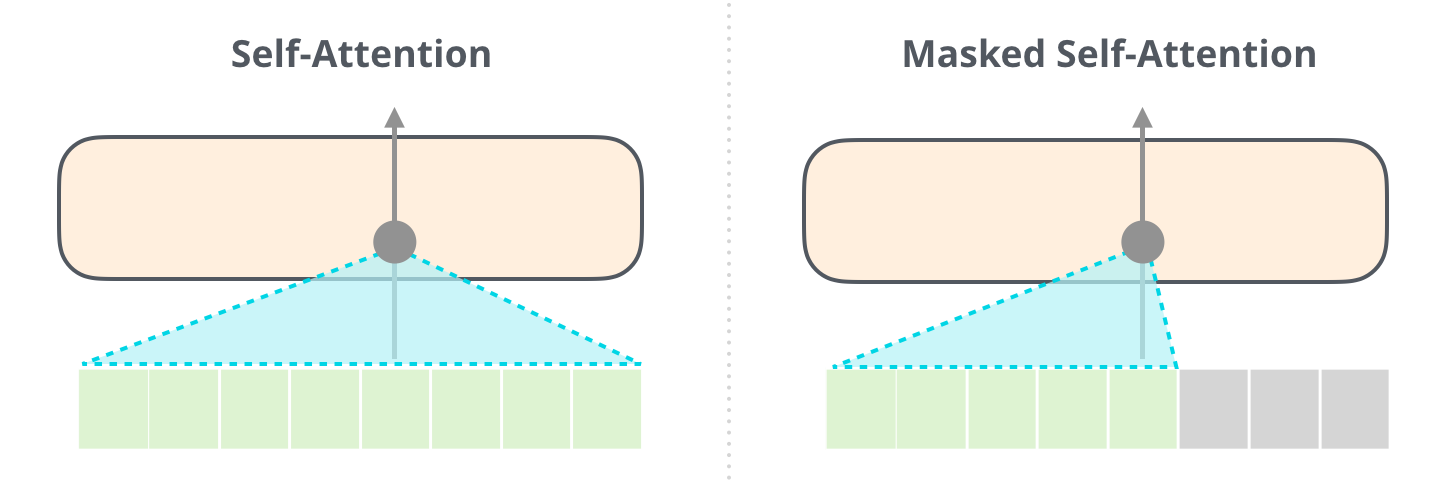### ordered input tokes

• attention to the left only (causality)
• value, key, query
$\mathbf{V}_m = \hat{V}_m \cdot \mathbf{x}_m, \qquad \mathbf{K}_m = \hat{K}_m \cdot \mathbf{x}_m$
$\mathbf{Q}_m = \hat{Q}_m \cdot \mathbf{x}_m$
• linearized attention
$$\begin{array}{rcl} \mathbf{s}_q^{\mathrm{(head)}} &= & \sum_k \mathbf{V}_k\, \mathrm{softmax}\Big(F_a(\mathbf{K}_k,\mathbf{Q}_{q})\Big) \\[0.5ex] &\to& \frac{\sum_k\mathbf{V}_k \big(\mathbf{K}_k\,\cdot\,\mathbf{Q}_{q}\big)} {\sum_k \mathbf{K}_k\,\cdot\,\mathbf{Q}_{q}} = \frac{\big(\sum_k \mathbf{V}_k \otimes\mathbf{K}_k\big)\,\cdot\,\mathbf{Q}_{q}} {\big(\sum_k \mathbf{K}_k\big)\,\cdot\,\mathbf{Q}_{q}} \end{array}$$
• outer product: $\ \otimes$

### associative soft weight computing

• enters linearized attention (mean-field approximation)
:: $\ W_{\rm tot}=\sum_k \mathbf{V}_k \otimes\mathbf{K}_k$
$$W_m = W_{m-1} + \mathbf{V}_m \otimes\mathbf{K}_m$$
• akin to time decomposition of a autoassociative recurrent net
:: internal state: $\ W_m$
• key-value associations
$$\mathbf{V}_m \otimes\mathbf{K}_m = \mathbf{x}_m\,\cdot\,\big(\hat{V}_m \otimes\hat{K}_m \big)\,\cdot\,\mathbf{x_m}$$

# why does it work?### attention in neuroscience / psychology

• external (saliency, 'something fast')
• top-down attention commands
:: 'looking for a red object'
$\color{brown}\Rightarrow \$ humunculus problem
• “No one knows what attention is”   [Hommel et al., 2019]

### ML attention

active during training $\ \color{brown}\Rightarrow\$ self-consitency loop

• Q, K, V matrices adapt to Q-K matching condition
:: dot product
• attention $\ \color{brown}\equiv\$ information routing
:: self-optimized information bootleneck
self-optimized information routing
solves the fitting / generalization dilemma
• statistical learning of causal relations
:: not (only) of information

# foundation models

### GPT - generative pretrained transformer

• number of adaptable parameters (matrix elements)
:: $10^8$ / $10^9$ / $10^{11}$ / $10^{12}$
:: GPT 1 / 2 / 3 / 4
• $10^3-10^4\$ context tokens, $\ \sim 80\$ layers
automatic pretraining by predicting next word
$\begin{array}{rl} {\color{red}\equiv} & \mathrm{base\ mode\ (GPT\!-\!3)} \\[0.0ex] {\color{red}+} & \mathrm{human\ supervised\ finetuning\ (SFT\ model)} \\[0.0ex] {\color{red}+} & \mathrm{human\ supervised\ reinforement\ learning} \\[0.0ex] {\color{red}\Rightarrow} & \mathrm{chat\ assistent\ (foundation\ model)} \end{array}$

• SFT: $\ 10^4\!-\!10^5\$ hand-crafted (prompt, response) pairs
:: learns to answer; ¬(text completion)

### applications / tasks on top of foundation model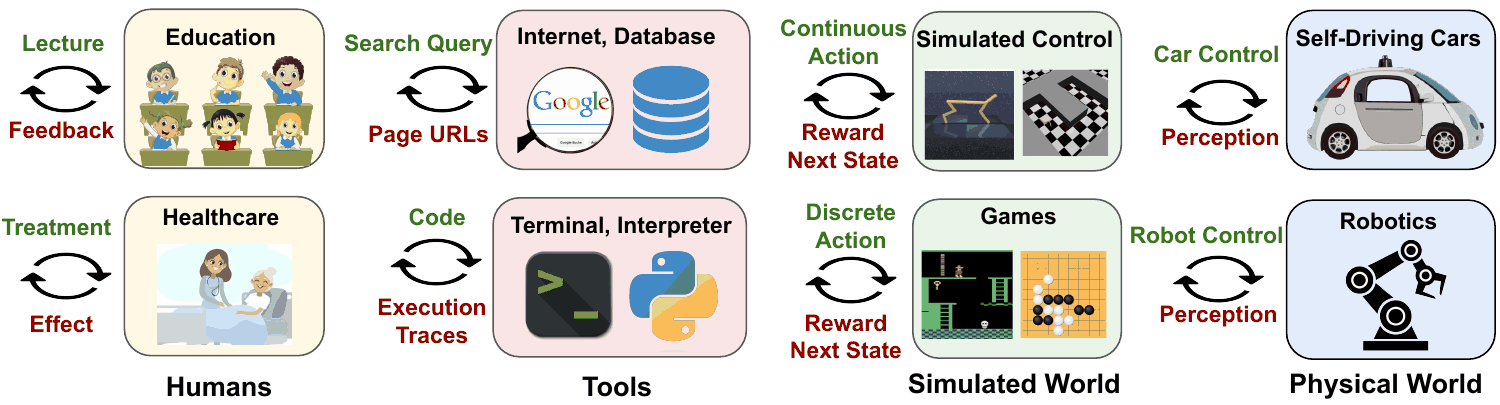# value alignment

### reinforcement learning from human feedback (RLHF)

• reinforcement learning
:: learning from feedback
• humans train the reward model
the reward model trains GPT
:: circumvents behavioral cloning
$\color{brown}\Rightarrow\$ value alignment
$\color{brown}\Rightarrow\$ ChatGPT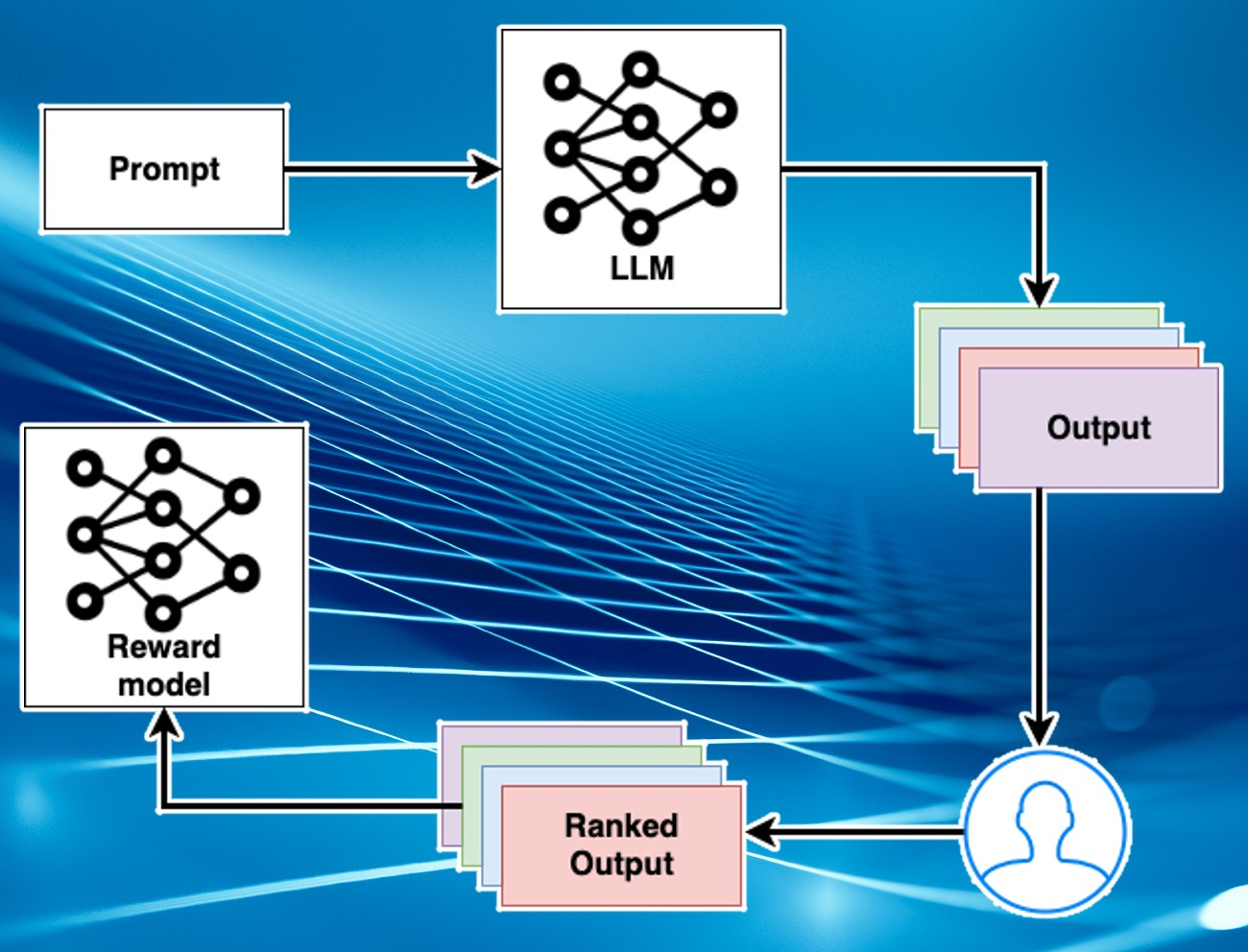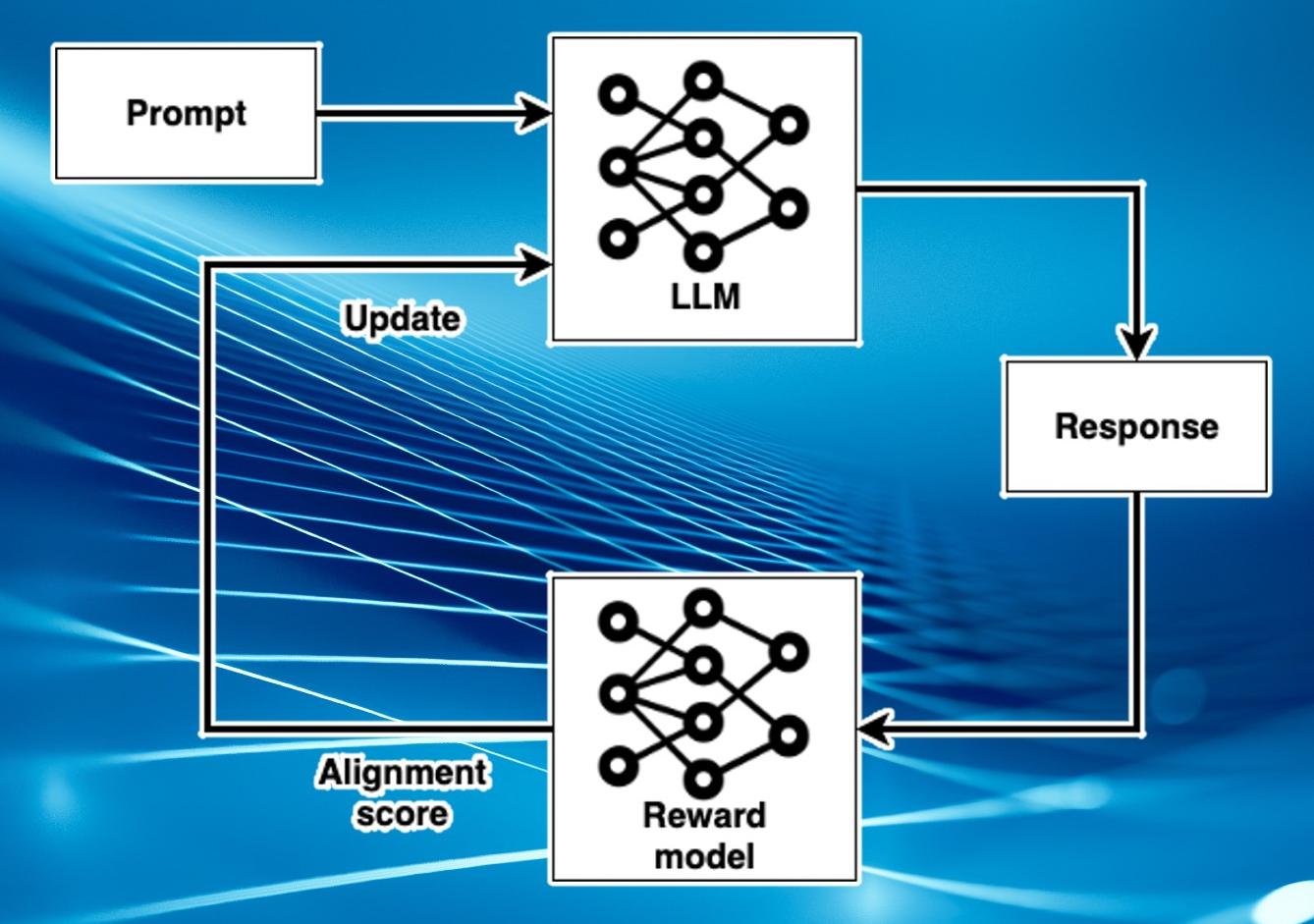# open-source explosion

### LLaMA (Meta AI)

• public release Feb 2023
:: 7B, 13B, 33B, and 65B parameters
:: parameters leaked within a week

### 'We have no moat'

• leaked Google memo, May 2023

### regulation

• data privacy / age verification / risk of wrong answers (Italy)
• EU AI act (dangerous applications)
• however, open-source explosion
:: regulation no possible?